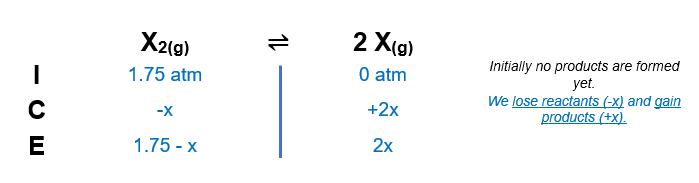# Problem: The reaction X2(g) ⇌ 2X(g) occurs in a closed reaction vessel at constant volume and temperature. Initially, the vessel contains only X2 at a pressure of 1.75 atm. After the reaction reaches equilibrium, the total pressure is 2.75 atm.What is the value of the equilibrium constant, Kp, for the reaction?

###### FREE Expert Solution
81% (272 ratings)
###### FREE Expert Solution

We are being asked to calculate the equilibrium constant, Kp for the given equilibrium reaction:

X2(g) ⇌ 2X(g)

When dealing with equilibrium and Kp:

Kp→ equilibrium units are in terms of pressure
Kp is an equilibrium expression:

$\overline{){{\mathbf{K}}}_{{\mathbf{p}}}{\mathbf{=}}\frac{\mathbf{products}}{\mathbf{reactants}}}$

only gaseous species are included in the equilibrium expression
▪ the coefficient of each compound in the reaction equation will be the exponent of the concentrations in the equilibrium expression

Given:

Initial Concentrations:

PX2 = 1.75 atm
PX = 0 atm

In order to calculate the equilibrium concentrations of species, we need to use an ICE ChartGiven values at equilibrium:

Ptotal = 2.75 atm

Calculate x:

x = 1.00 atm

We can now calculate the equilibrium constant, Kp:

X2(g) ⇌ 2X(g)

81% (272 ratings)###### Problem Details

The reaction X2(g) ⇌ 2X(g) occurs in a closed reaction vessel at constant volume and temperature. Initially, the vessel contains only X2 at a pressure of 1.75 atm. After the reaction reaches equilibrium, the total pressure is 2.75 atm.

What is the value of the equilibrium constant, Kp, for the reaction?

What scientific concept do you need to know in order to solve this problem?

Our tutors have indicated that to solve this problem you will need to apply the ICE Chart concept. You can view video lessons to learn ICE Chart. Or if you need more ICE Chart practice, you can also practice ICE Chart practice problems.

What is the difficulty of this problem?

Our tutors rated the difficulty ofThe reaction X2(g) ⇌ 2X(g) occurs in a closed reaction vesse...as high difficulty.

How long does this problem take to solve?

Our expert Chemistry tutor, Dasha took 4 minutes and 49 seconds to solve this problem. You can follow their steps in the video explanation above.

What professor is this problem relevant for?

Based on our data, we think this problem is relevant for Professor Siler's class at OREGONSTATE.